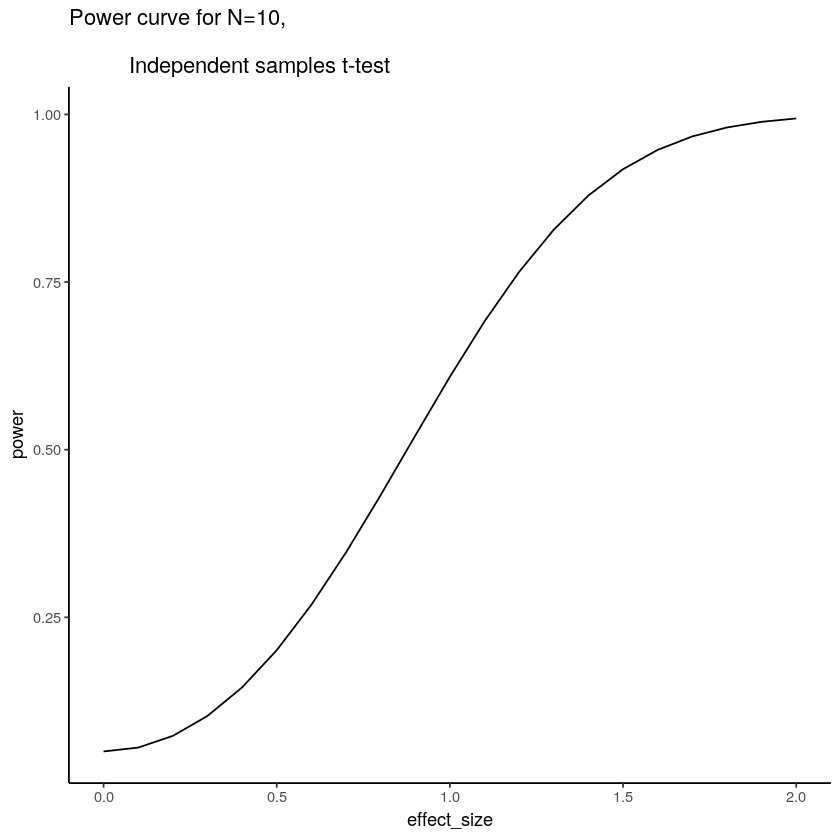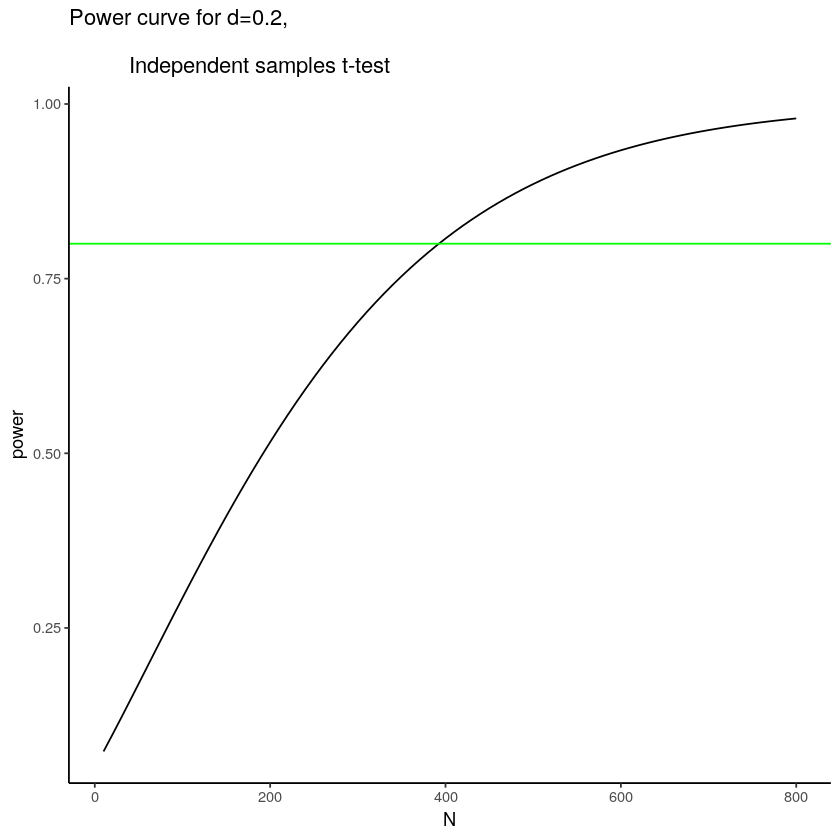# 12.2: Power

$$\newcommand{\vecs}{\overset { \rightharpoonup} {\mathbf{#1}} }$$ $$\newcommand{\vecd}{\overset{-\!-\!\rightharpoonup}{\vphantom{a}\smash {#1}}}$$$$\newcommand{\id}{\mathrm{id}}$$ $$\newcommand{\Span}{\mathrm{span}}$$ $$\newcommand{\kernel}{\mathrm{null}\,}$$ $$\newcommand{\range}{\mathrm{range}\,}$$ $$\newcommand{\RealPart}{\mathrm{Re}}$$ $$\newcommand{\ImaginaryPart}{\mathrm{Im}}$$ $$\newcommand{\Argument}{\mathrm{Arg}}$$ $$\newcommand{\norm}{\| #1 \|}$$ $$\newcommand{\inner}{\langle #1, #2 \rangle}$$ $$\newcommand{\Span}{\mathrm{span}}$$ $$\newcommand{\id}{\mathrm{id}}$$ $$\newcommand{\Span}{\mathrm{span}}$$ $$\newcommand{\kernel}{\mathrm{null}\,}$$ $$\newcommand{\range}{\mathrm{range}\,}$$ $$\newcommand{\RealPart}{\mathrm{Re}}$$ $$\newcommand{\ImaginaryPart}{\mathrm{Im}}$$ $$\newcommand{\Argument}{\mathrm{Arg}}$$ $$\newcommand{\norm}{\| #1 \|}$$ $$\newcommand{\inner}{\langle #1, #2 \rangle}$$ $$\newcommand{\Span}{\mathrm{span}}$$$$\newcommand{\AA}{\unicode[.8,0]{x212B}}$$

When there is a true effect out there to measure, you want to make sure your design is sensitive enough to detect the effect, otherwise what’s the point. We’ve already talked about the idea that an effect can have different sizes. The next idea is that your design can be more less sensitive in its ability to reliabily measure the effect. We have discussed this general idea many times already in the textbook, for example we know that we will be more likely to detect “significant” effects (when there are real differences) when we increase our sample-size. Here, we will talk about the idea of design sensitivity in terms of the concept of power. Interestingly, the concept of power is a somewhat limited concept, in that it only exists as a concept within some philosophies of statistics.

## A digresssion about hypothesis testing

In particular, the concept of power falls out of the Neyman-Pearson concept of null vs. alternative hypothesis testing. Up to this point, we have largely avoided this terminology. This is perhaps a disservice in that the Neyman-Pearson ideas are by now the most common and widespread, and in the opinion of some of us, they are also the most widely misunderstood and abused idea, which is why we have avoided these ideas until now.

What we have been mainly doing is talking about hypothesis testing from the Fisherian (Sir Ronald Fisher, the ANOVA guy) perspective. This is a basic perspective that we think can’t be easily ignored. It is also quite limited. The basic idea is this:

1. We know that chance can cause some differences when we measure something between experimental conditions.
2. We want to rule out the possibility that the difference that we observed can not be due to chance
3. We construct large N designs that permit us to do this when a real effect is observed, such that we can confidently say that big differences that we find are so big (well outside the chance window) that it is highly implausible that chance alone could have produced.
4. The final conclusion is that chance was extremely unlikely to have produced the differences. We then infer that something else, like the manipulation, must have caused the difference.
5. We don’t say anything else about the something else.
6. We either reject the null distribution as an explanation (that chance couldn’t have done it), or retain the null (admit that chance could have done it, and if it did we couldn’t tell the difference between what we found and what chance could do)

Neyman and Pearson introduced one more idea to this mix, the idea of an alternative hypothesis. The alternative hypothesis is the idea that if there is a true effect, then the data sampled into each condition of the experiment must have come from two different distributions. Remember, when there is no effect we assume all of the data cam from the same distribution (which by definition can’t produce true differences in the long run, because all of the numbers are coming from the same distribution). The graphs of effect-sizes from before show examples of these alternative distributions, with samples for condition A coming from one distribution, and samples from condition B coming from a shifted distribution with a different mean.

So, under the Neyman-Pearson tradition, when a researcher find a signifcant effect they do more than one things. First, they reject the null-hypothesis of no differences, and they accept the alternative hypothesis that there was differences. This seems like a sensible thing to do. And, because the researcher is actually interested in the properties of the real effect, they might be interested in learning more about the actual alternative hypothesis, that is they might want to know if their data come from two different distributions that were separated by some amount…in other words, they would want to know the size of the effect that they were measuring.

## Back to power

We have now discussed enough ideas to formalize the concept of statistical power. For this concept to exist we need to do a couple things.

1. Agree to set an alpha criterion. When the p-value for our test-statistic is below this value we will call our finding statistically significant, and agree to reject the null hypothesis and accept the “alternative” hypothesis (sidenote, usually it isn’t very clear which specific alternative hypothesis was accepted)
2. In advance of conducting the study, figure out what kinds of effect-sizes our design is capable of detecting with particular probabilites.

The power of a study is determined by the relationship between

1. The sample-size of the study
2. The effect-size of the manipulation
3. The alpha value set by the researcher.

To see this in practice let’s do a simulation. We will do a t-test on a between-groups design 10 subjects in each group. Group A will be a control group with scores sampled from a normal distribution with mean of 10, and standard deviation of 5. Group B will be a treatment group, we will say the treatment has an effect-size of Cohen’s $$d$$ = .5, that’s a standard deviation shift of .5, so the scores with come from a normal distribution with mean =12.5 and standard deivation of 5. Remember 1 standard deviation here is 5, so half of a standard deviation is 2.5.

The following R script runs this simulated experiment 1000 times. We set the alpha criterion to .05, this means we will reject the null whenever the $$p$$-value is less than .05. With this specific design, how many times out of of 1000 do we reject the null, and accept the alternative hypothesis?

p<-length(1000)
for(i in 1:1000){
A<-rnorm(10,10,5)
B<-rnorm(10,12.5,5)
p[i]<-t.test(A,B,var.equal = TRUE)$p.value } length(p[p<.05]) 179 The answer is that we reject the null, and accept the alternative 179 times out of 1000. In other words our experiment succesfully accepts the alternative hypothesis 17.9 percent of the time, this is known as the power of the study. Power is the probability that a design will succesfully detect an effect of a specific size. Importantly, power is completely abstract idea that is completely determined by many assumptions including N, effect-size, and alpha. As a result, it is best not to think of power as a single number, but instead as a family of numbers. For example, power is different when we change N. If we increase N, our samples will more precisely estimate the true distributions that they came from. Increasing N reduces sampling error, and shrinks the range of differences that can be produced by chance. Lets’ increase our N in this simulation from 10 to 20 in each group and see what happens. p<-length(1000) for(i in 1:1000){ A<-rnorm(20,10,5) B<-rnorm(20,12.5,5) p[i]<-t.test(A,B,var.equal = TRUE)$p.value
}
length(p[p<.05])
360

Now the number of significant experiments is 360 out of 1000, or a power of 36 percent. That’s roughly doubled from before. We have made the design more sensitive to the effect by increasing N.

We can change the power of the design by changing the alpha-value, which tells us how much evidence we need to reject the null. For example, if we set the alpha criterion to 0.01, then we will be more conservative, only rejecting the null when chance can produce the observed difference 1% of the time. In our example, this will have the effect of reducing power. Let’s keep N at 20, but reduce the alpha to 0.01 and see what happens:

p<-length(1000)
for(i in 1:1000){
A<-rnorm(20,10,5)
B<-rnorm(20,12.5,5)
p[i]<-t.test(A,B,var.equal = TRUE)$p.value } length(p[p<.01]) 138 Now only 138 out of 1000 experiments are significant, that’s 13.8 power. Finally, the power of the design depends on the actual size of the effect caused by the manipulation. In our example, we hypothesized that the effect caused a shift of .5 standard deviations. What if the effect causes a bigger shift? Say, a shift of 2 standard deviations. Let’s keep N= 20, and alpha < .01, but change the effect-size to two standard deviations. When the effect in the real-world is bigger, it should be easier to measure, so our power will increase. p<-length(1000) for(i in 1:1000){ A<-rnorm(20,10,5) B<-rnorm(20,30,5) p[i]<-t.test(A,B,var.equal = TRUE)$p.value
}
length(p[p<.01])
1000

Neat, if the effect-size is actually huge (2 standard deviation shift), then we have power 100 percent to detect the true effect.

## Power curves

We mentioned that it is best to think of power as a family of numbers, rather than as a single number. To elaborate on this consider the power curve below. This is the power curve for a specific design: a between groups experiments with two levels, that uses an independent samples t-test to test whether an observed difference is due to chance. Critically, N is set to 10 in each group, and alpha is set to .05

Power (as a proportion, not a percentage) is plotted on the y-axis, and effect-size (Cohen’s d) in standard deviation units is plotted on the x-axis.Figure $$\PageIndex{1}$$: This figure shows power as a function of effect-size (Cohen’s d) for a between-subjects independent samples t-test, with N=10, and alpha criterion 0.05.

A power curve like this one is very helpful to understand the sensitivity of a particular design. For example, we can see that a between subjects design with N=10 in both groups, will detect an effect of d=.5 (half a standard deviation shift) about 20% of the time, will detect an effect of d=.8 about 50% of the time, and will detect an effect of d=2 about 100% of the time. All of the percentages reflect the power of the design, which is the percentage of times the design would be expected to find a $$p$$ < 0.05.

Let’s imagine that based on prior research, the effect you are interested in measuring is fairly small, d=0.2. If you want to run an experiment that will detect an effect of this size a large percentage of the time, how many subjects do you need to have in each group? We know from the above graph that with N=10, power is very low to detect an effect of d=0.2. Let’s make another graph, but vary the number of subjects rather than the size of the effect.Figure $$\PageIndex{2}$$: This figure shows power as a function of N for a between-subjects independent samples t-test, with d=0.2, and alpha criterion 0.05.

The figure plots power to detect an effect of d=0.2, as a function of N. The green line shows where power = .8, or 80%. It looks like we would nee about 380 subjects in each group to measure an effect of d=0.2, with power = .8. This means that 80% of our experiments would succesfully show p < 0.05. Often times power of 80% is recommended as a reasonable level of power, however even when your design has power = 80%, your experiment will still fail to find an effect (associated with that level of power) 20% of the time!

This page titled 12.2: Power is shared under a CC BY-SA 4.0 license and was authored, remixed, and/or curated by Matthew J. C. Crump via source content that was edited to the style and standards of the LibreTexts platform; a detailed edit history is available upon request.